Courses

# Test: Electromagnetic Induction - From Past 28 Years Questions

## 25 Questions MCQ Test Physics Class 12 | Test: Electromagnetic Induction - From Past 28 Years Questions

Description
This mock test of Test: Electromagnetic Induction - From Past 28 Years Questions for Class 12 helps you for every Class 12 entrance exam. This contains 25 Multiple Choice Questions for Class 12 Test: Electromagnetic Induction - From Past 28 Years Questions (mcq) to study with solutions a complete question bank. The solved questions answers in this Test: Electromagnetic Induction - From Past 28 Years Questions quiz give you a good mix of easy questions and tough questions. Class 12 students definitely take this Test: Electromagnetic Induction - From Past 28 Years Questions exercise for a better result in the exam. You can find other Test: Electromagnetic Induction - From Past 28 Years Questions extra questions, long questions & short questions for Class 12 on EduRev as well by searching above.
QUESTION: 1

### An inductor may store energy in          

Solution:

An inductor stores energy in its magnetic field.

QUESTION: 2

### The current in self inductance L = 40 mH is to beincreased uniformly from 1 amp to 11 amp in 4 milliseconds. The e.m.f. induced in the inductorduring the process is    

Solution: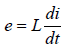Given that L = 40 × 10–3 H,
di = 11 A – 1 A = 10 A
and dt = 4 × 10–3 s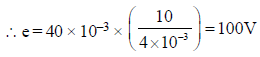QUESTION: 3

### A magnetic field of 2 × 10–2 T acts at right anglesto a coil of area 100 cm2, with 50 turns. Theaverage e.m.f. induced in the coil is 0.1 V, when itis removed from the field in t sec. The value of t is

Solution: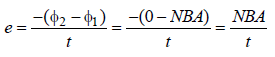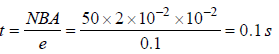QUESTION: 4

A 100 millihenry coil carries a current of 1A.Energy stored in its magnetic field is 

Solution: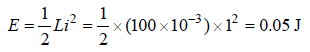QUESTION: 5

If the number of turns per unit length of a coil ofsolenoid is doubled, the self-inductance of thesolenoid will 

Solution:

Self inductance of a solenoid =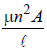So, self induction ∝ n2
So, inductance becomes 4 times when n is doubled.

QUESTION: 6

A rectangular coil of 20 turns and area of crosssection 25 sq. cm has a resistance of 100Ω. If amagnetic field which is perpendicular to the planeof coil changes at a rate of 1000 tesla per second,the current in the coil is 

Solution: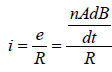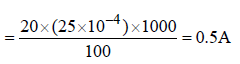QUESTION: 7

The total charge induced in a conducting loopwhen it is moved in a magnetic field depend on         

Solution: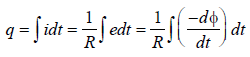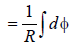(taking only magnitude of e)
Hence, total charge induced in the
conducting loop depends upon the total
change in magnetic flux.

QUESTION: 8

What is the self-inductance of a coil whichproduces 5V when the current changes from 3ampere to 2 ampere in one millisecond?       

Solution: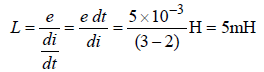QUESTION: 9

If N is the number of turns in a coil, the value ofself inductance varies as 

Solution: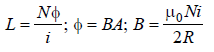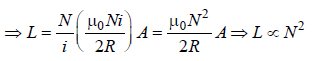QUESTION: 10

A conductor of length 0.4 m is moving with aspeed of 7 m/s perpendicular to a magnetic fieldof intensity 0.9 Wb/m2. The induced e.m.f.across the conductor is               

Solution:

Length of conductor (l) = 0.4 m; Speed (v) = 7 m/s and magnetic field (B) = 0.9 Wb/ m2.
Induced e.m.f. (V) = Blv sin θ = 0.9 × 0.4 × 7
× sin 90º = 2.52 V.

QUESTION: 11

A varying current in a coil changes from 10A tozero in 0.5 sec. If the average e.m.f induced inthe coil is 220V, the self-inductance of the coil is              

Solution:

Initial current (I1) = 10 A; Final current
(I2)= 0; Time (t) = 0.5 sec and induced e.m.f.
(ε) = 220 V.
Induced e.m.f. (ε)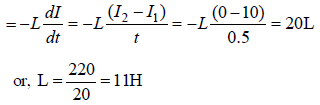[where L = Self inductance of coil]

QUESTION: 12

Two coils have a mutual inductance 0.005 H.The current changes in the first coil accordingto equation I = I0 sin ωt, where I0 = 10A and ω = 100π radian/sec. The maximum value of e.m.f.in the second coil is 

Solution: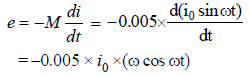emax . = 0.005x i0 xω (when cos ωt = –1)
= 0.005 × 10 × 100π = 5πV

QUESTION: 13

In an inductor of self-inductance L = 2 mH,current changes with time according to relationi = t2e–t. At what time emf is zero?              

Solution:

L = 2mH, i = t2e–t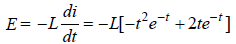when E = 0,
–e–t t2 + 2te–t = 0
or, 2t e–t = e–t t2
⇒t = 2 sec.

QUESTION: 14

The magnetic flux through a circuit of resistance R changes by an amount Δφ in a time Δt. Then the total quantity of electric charge Q that passes any point in the circuit during the time Δt is represented by        

Solution: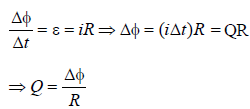QUESTION: 15

As a result of change in the magnetic flux linkedto the closed loop shown in the Fig,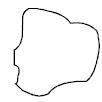an e.m.f. V volt is induced in the loop. The work done (joules) in taking a charge Q coulomb once  along the loop is



Solution: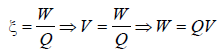QUESTION: 16

Two coils of self inductances 2 mH and 8 mH areplaced so close together that the effective fluxin one coil is completely linked with the other.The mutual inductance between these coils is              

Solution:

Mutual Inductance of two coils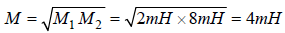QUESTION: 17

A long solenoid has 500 turns. When a currentof 2 ampere is passed through it, the resultingmagnetic flux linked with each turn of thesolenoid is 4 ×10–3 Wb. The self- inductance ofthe solenoid is        

Solution:

Total number of turns in the solenoid,
N = 500
Current, I = 2A.
Magnetic flux linked with each turn
= 4 × 10–3 Wb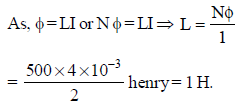QUESTION: 18

A rectangular, a square, a circular and an elliptical loop, all in the (x – y) plane, are moving out of a uniform magnetic field with a constant velocity,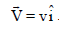The magnetic field is directed along the negative z axis direction. The induced emf, during the passage of these loops, out of the field region, will not remain constant for      

Solution:

The induced emf will remain constant only in the case of rectangular and square loops. In case of the circular and the elliptical loops, the rate of change of area of the loops during their passage out of the field is not constant, hence induced emf will not remain constant for them.

QUESTION: 19

A conducting circular loop is placed in a uniformmagnetic field of 0.04 T with its planeperpendicular to the magnetic field. The radiusof the loop starts shrinking at 2 mm/s. The induced emf in the loop when the radius is 2 cmis 

Solution:

Induced emf in the loop is given by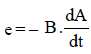where A is the area of the loop.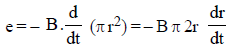r = 2cm = 2 × 10–2 m
dr = 2 mm = 2 × 10–3 m
dt = 1s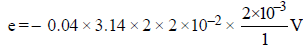= 0.32 π × 10–5V
= 3.2 π ×10–6V
= 3.2 πμV

QUESTION: 20

A conducting circular loop is placed in a uniform magnetic field, B = 0.025 T with its plane perpendicular to the loop. The radius of the loop is made to shrink at a constant rate of 1 mm s–1. The induced e.m.f. when the radius is 2 cm, is                             

Solution:

Magnetic flux linked with the loop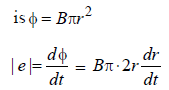When r = 2 cm, dr/dt = 1 mms-1

e = 0.025× π×2 ×2 ×10–2×10–3
= 0.100 × π× 10–5 = π × 10–6 V = πμV

QUESTION: 21

The current i in a coil varies with time as shown in the figure. The variation of induced emf with time would be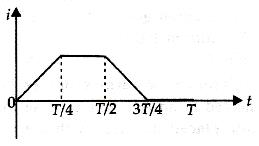Solution: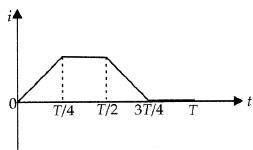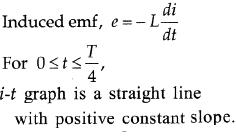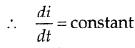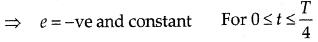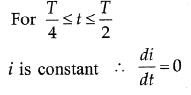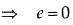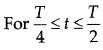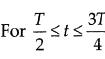i-t graph is a straight line with negative constant slope.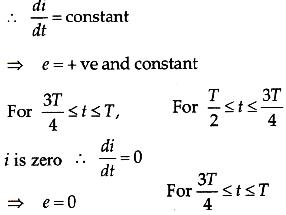From this analysis, the variation of induced emf with time as shown in the figure below.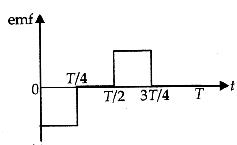QUESTION: 22

A coil of resistance 400Ω is placed in a magneticfield. If the magnetic flux φ(wb) linked with thecoil varies with time t (sec) as φ = 50t2 + 4. Thecurrent in the coil at t = 2 sec is : 

Solution:

According, to Faraday’s law of induction
Induced e.m.f.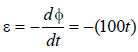Induced current i at t = 2 sec.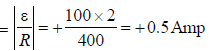QUESTION: 23

In a coil of resistance 10 Ω, the induced current developed by changing magnetic flux through it, is shown in figure as a function of time. The magnitude of change in flux through the coil in Weber is :          [2012M]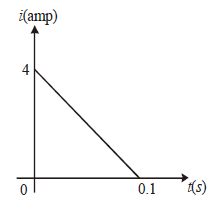Solution:

The charge through the coil = area of
current-time(i – t) graph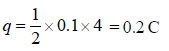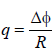∵ Change in flux (Δφ ) = q × R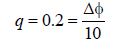Δφ = 2 Weber

QUESTION: 24

A wire loop is rotated in a magnetic field. Thefrequency of change of direction of the inducede.m.f. is [NEET 2013]

Solution:

This is the case of periodic EMI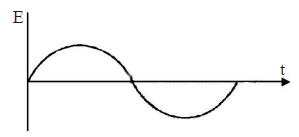From graph, it is clear that direction is
changing once in 1/2 cycle.

QUESTION: 25

A current of 2.5 A flows through a coil ofinductance 5 H. The magnetic flux linked withthe coil is [NEET Kar. 2013]

Solution:

Given: current I = 2.5 A
Inductance, L = 5H
Magnatic flux, φ=?
We know, φ = LI ⇒ 5 × 2.5 Wb = 12.5 Wb